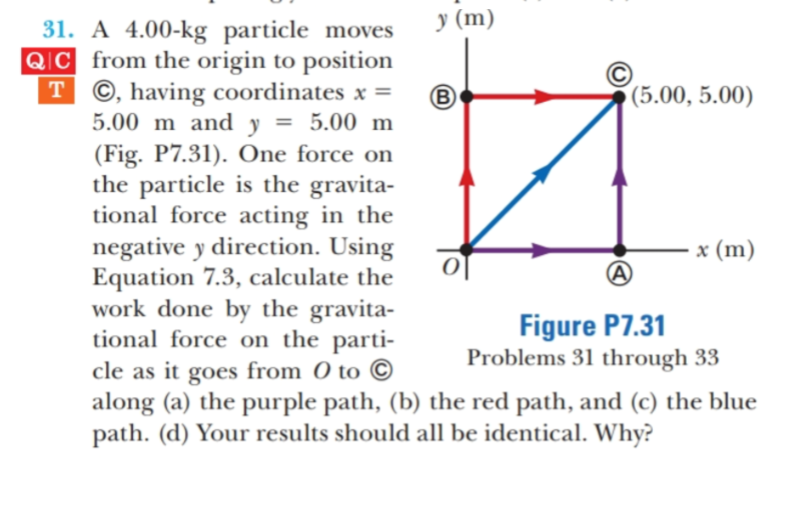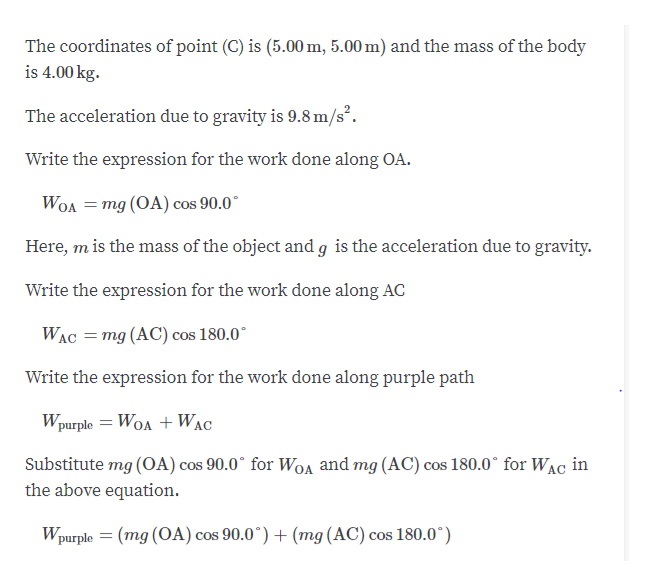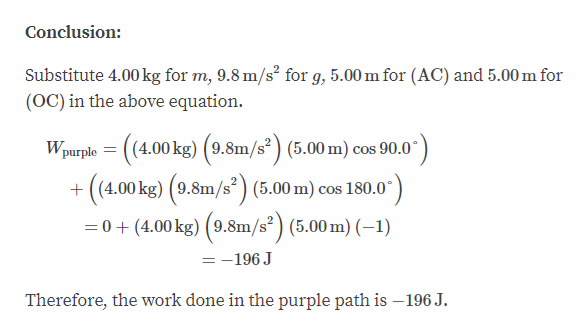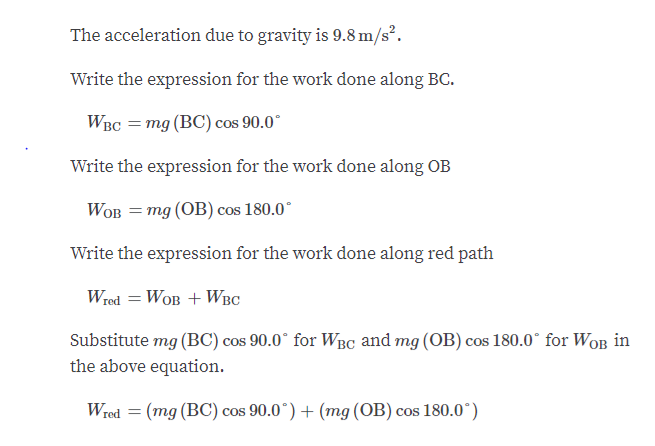# у (m)31. A 4.00-kg particle movesQIC from the origin to positionT ©, having coordinates x =5.00 m and y(Fig. P7.31). One force onthe particle is the gravita-tional force acting in thenegative y direction. UsingEquation 7.3, calculate thework done by the gravita-tional force on the parti-cle as it goes from O to Calong (a) the purple path, (b) the red path, and (c) the bluepath. (d) Your results should all be identical. Why?(5.00, 5.00)5.00 mx (m)Figure P7.31Problems 31 through 33

Questionhelp_outlineImage Transcriptioncloseу (m) 31. A 4.00-kg particle moves QIC from the origin to position T ©, having coordinates x = 5.00 m and y (Fig. P7.31). One force on the particle is the gravita- tional force acting in the negative y direction. Using Equation 7.3, calculate the work done by the gravita- tional force on the parti- cle as it goes from O to C along (a) the purple path, (b) the red path, and (c) the blue path. (d) Your results should all be identical. Why? (5.00, 5.00) 5.00 m x (m) Figure P7.31 Problems 31 through 33 fullscreen
check_circleExpert Solution
Step 1

Part A:help_outlineImage TranscriptioncloseThe coordinates of point (C) is (5.00 m, 5.00 m) and the mass of the body is 4.00 kg. The acceleration due to gravity is 9.8 m/s2 Write the expression for the work done along OA. WOA mg (OA) cos 90.0 COS Here, m is the mass of the object and g is the acceleration due to gravity. Write the expression for the work done along AC mg (AC) cos 180.0 WAC Write the expression for the work done along purple path Wpurple WOAWAC Substitute mg (OA) cos 90.0 for WoA and mg (AC) cos 180.0 for WAC in the above equation. Wpurple (mg (OA) cos 90.0 + (mg (AC) cos 180.0* fullscreen
Step 2help_outlineImage TranscriptioncloseConclusion: Substitute 4.00kg for m, 9.8 m/s2 for g, 5.00 m for (AC) and 5.00 m for (OC) in the above equation Wpurple ((4.00 kg) 9.8m/s2(5.00 m) cos 90.0 ((4.00 kg) 9.8m/s (5.00 m) cos 180.0 0(4.00kg) (9.8m/s2) (5.00 m) (-1) =-196 J Therefore, the work done in the purple path is -196 J. fullscreen
Step 3

Part B:

...help_outlineImage TranscriptioncloseThe acceleration due to gravity is 9.8 m/s2. Write the expression for the work done along BC. WBс 3 тg (ВC) сos 90.0" Write the expression for the work done along OB WOB = mg (OB) cos 180.0 Write the expression for the work done along red path Wred WoB WBC Substitute mg (BC) cos 90.0 for WBC and mg (OB) cos 180.0 for WoB in the above equation. (mg (BC) cos 90.0*) (mg (OB) cos 180.0° Wred fullscreen

### Want to see the full answer?

See Solution

#### Want to see this answer and more?

Solutions are written by subject experts who are available 24/7. Questions are typically answered within 1 hour*

See Solution
*Response times may vary by subject and question
Tagged in

### Work,Power and Energy